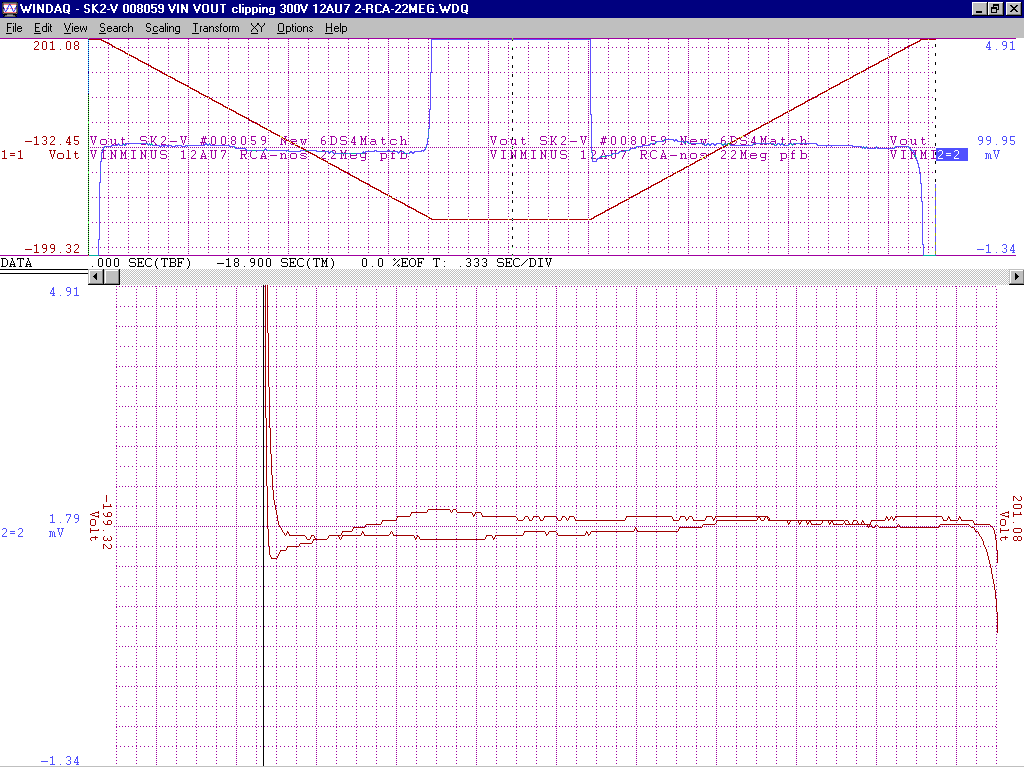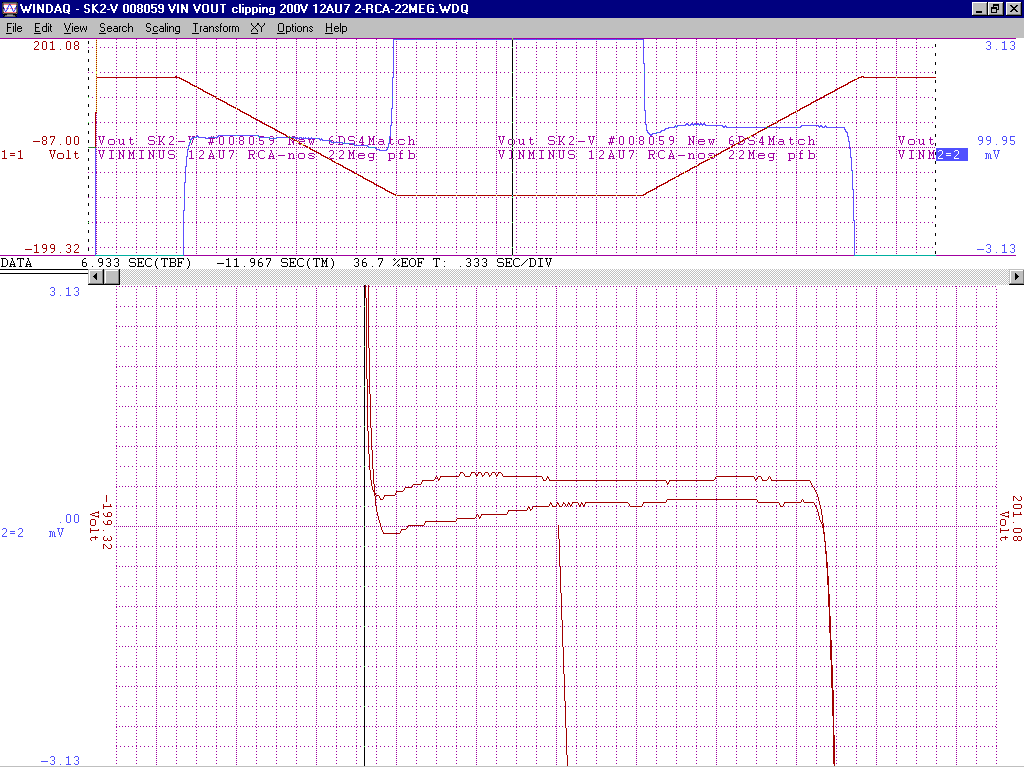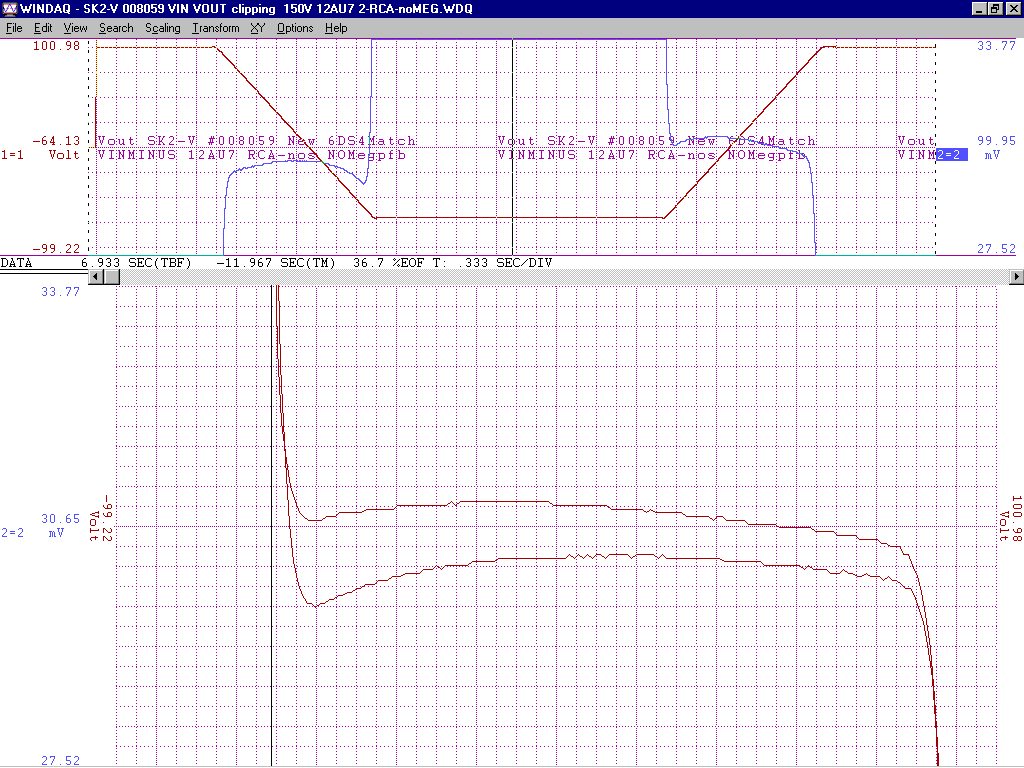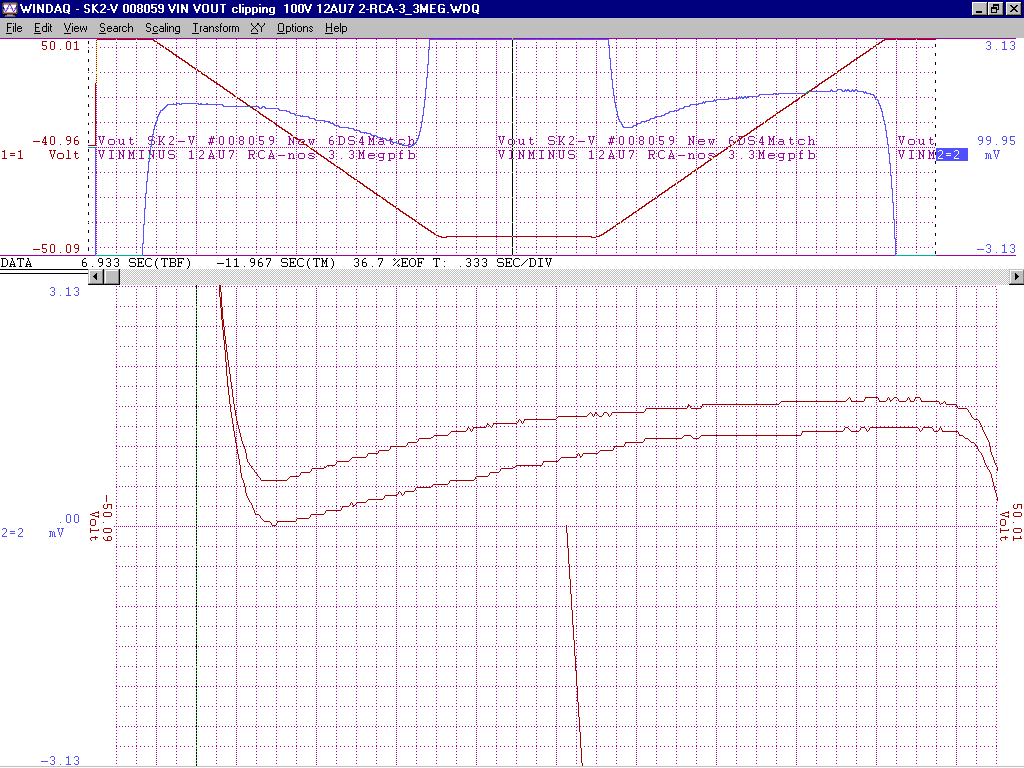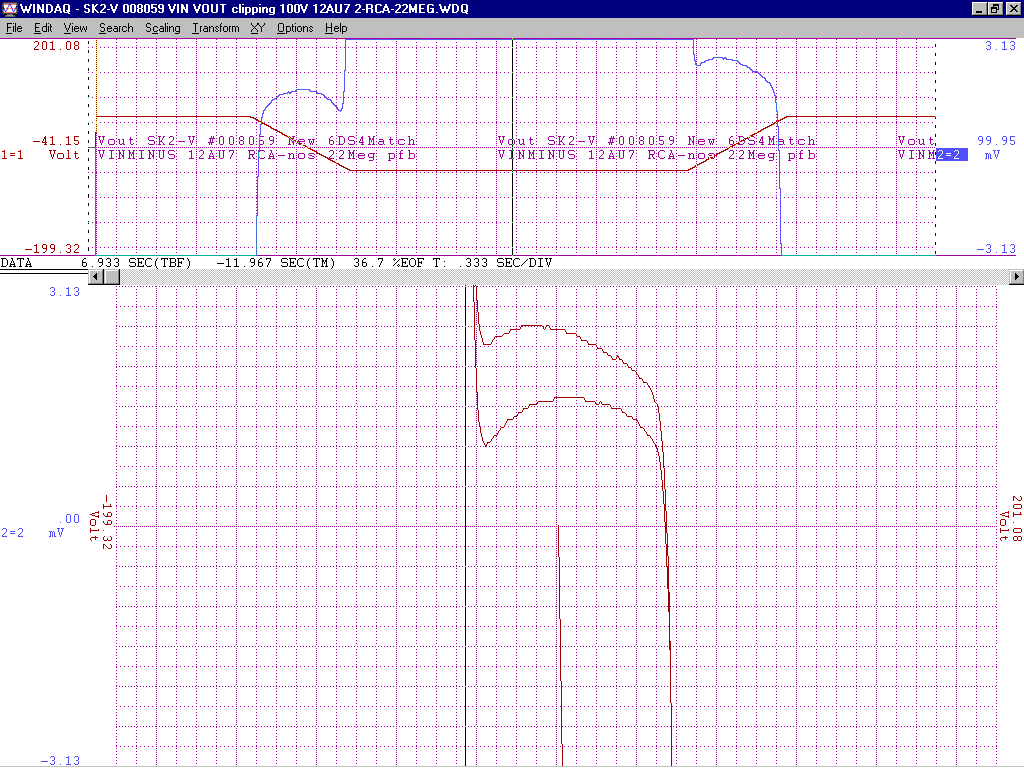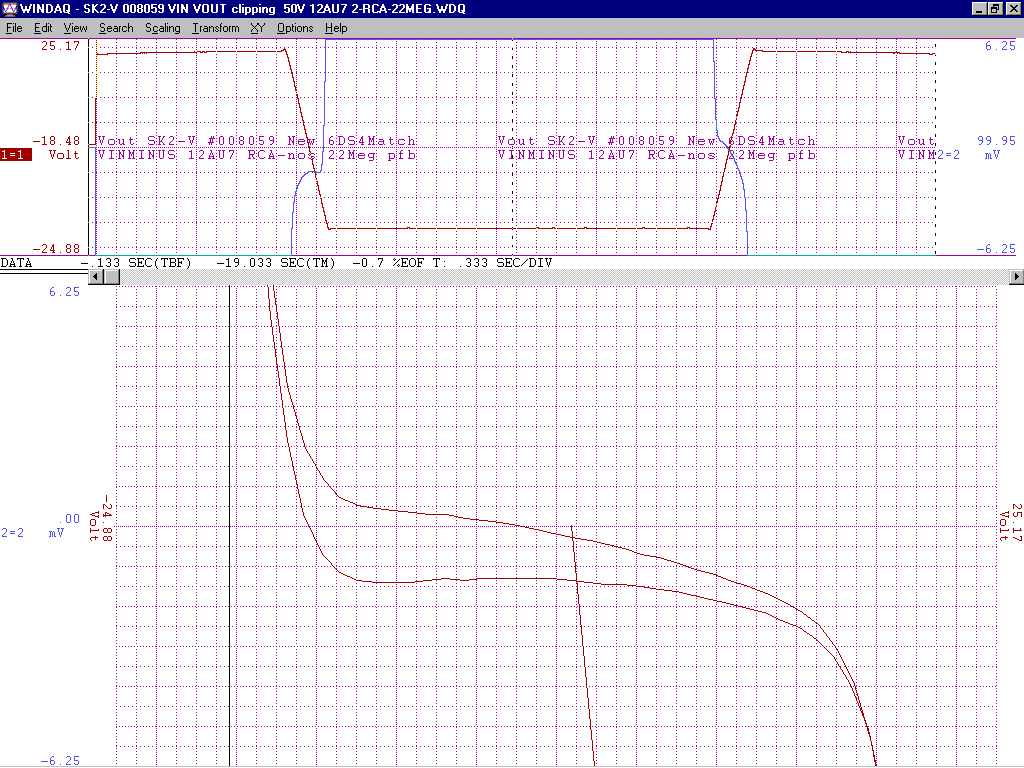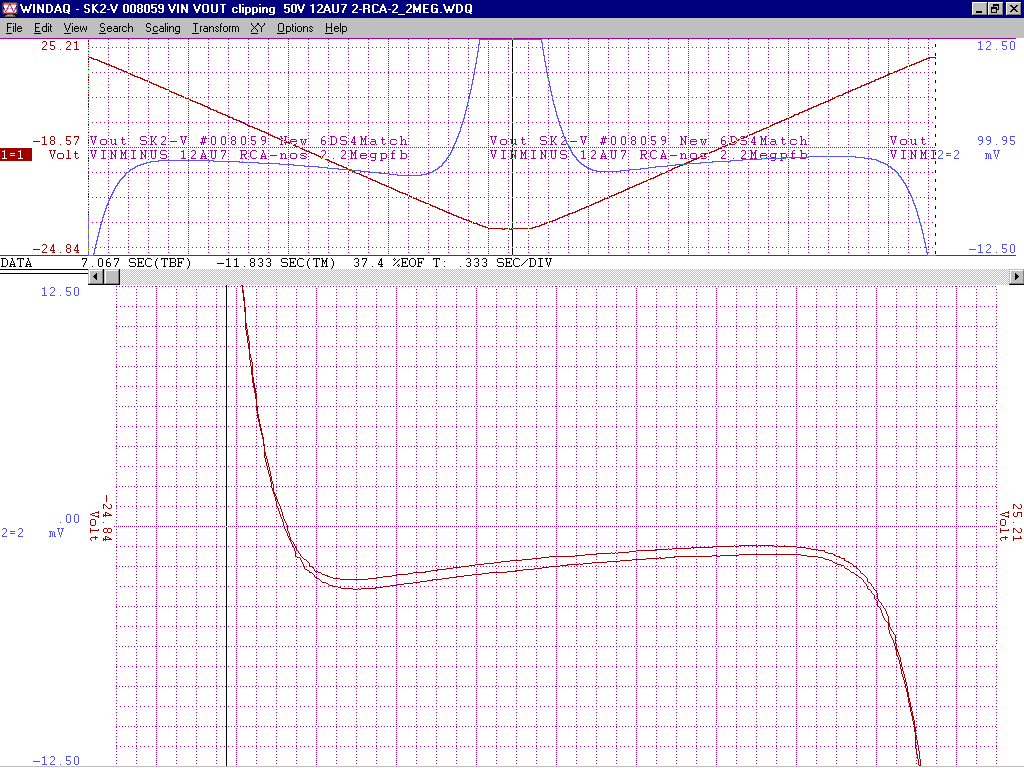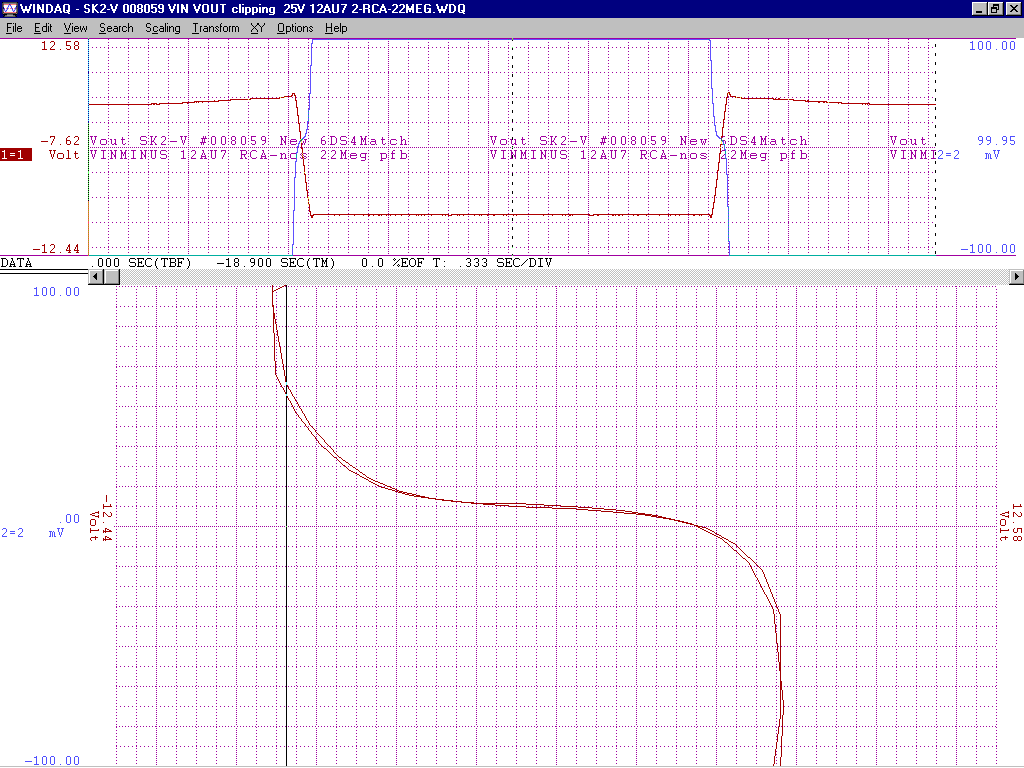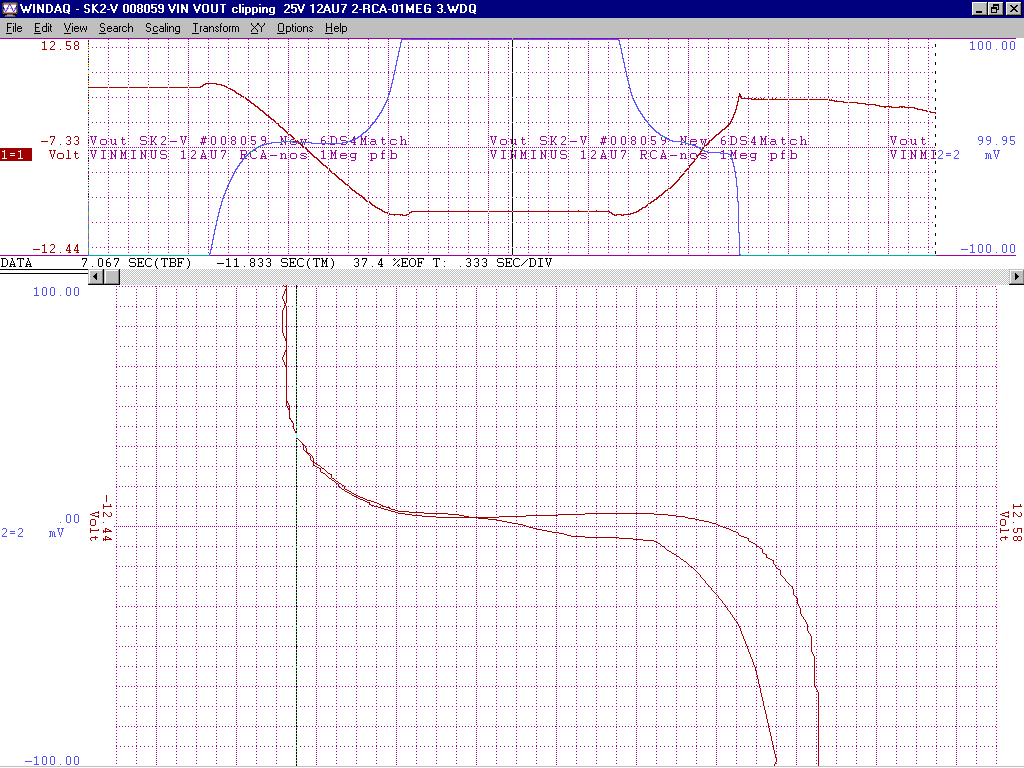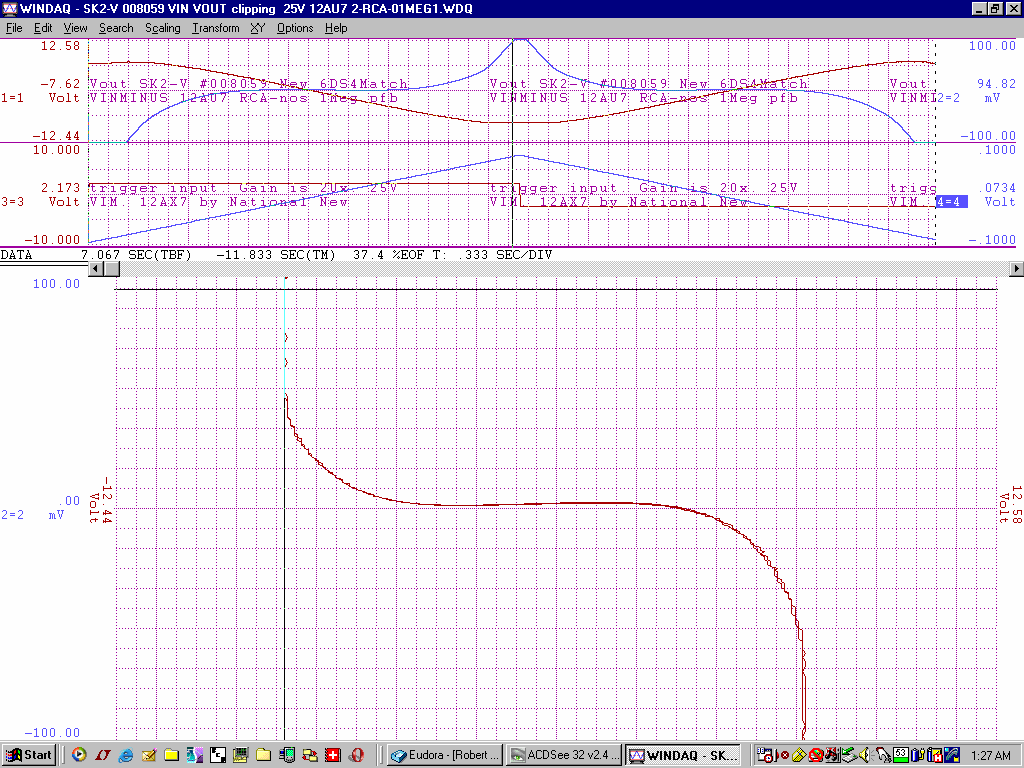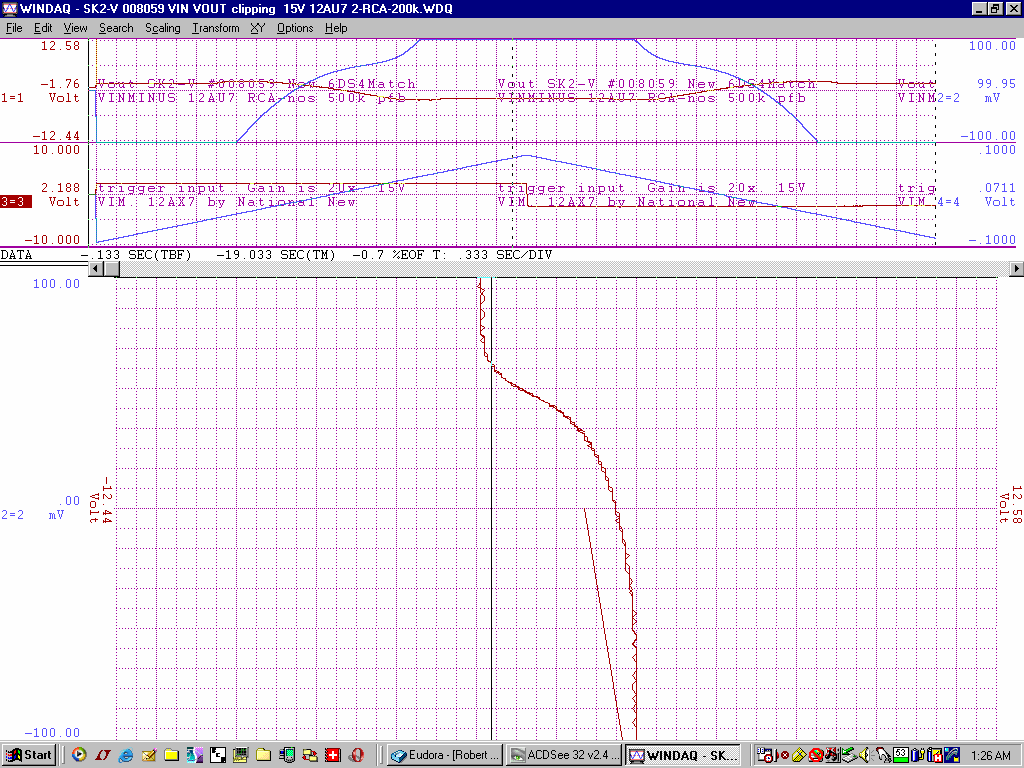+/-300V gain of about 1 million from -150V to +200V deltaV=350uVp-p

+/-250V gain of about 720k from -110V to +164V deltaV=390uVp-p

+/-200V gain of about 540k from -92V to +118V deltaV=390uVp-p

+/-150V gain of about 350k from -56V to +83V deltaV=390uVp-p

+/-100V gain of about 85k from -35V to +50V deltaV=1mVp-p

+/-50V  gain of about 15k from -15V to +15V deltaV=2mVp-p

+/-25V  gain of about 500 from -4V to +4V deltaV=16mVp-p

+/-25V  gain of about 1000 from -5V to +5V deltaV=10mVp-p with an additional 1Meg of pfb between the output 12AU7 cathodes

+/-20V  gain of about 500 from -3V to +1V deltaV=8mVp-p with an additional 500k of pfb  between the output 12AU7 cathodes

+/-15V  gain of about 100 from -2V to +1V deltaV=32mVp-p with an additional 200k of pfb between the output 12AU7 cathodes